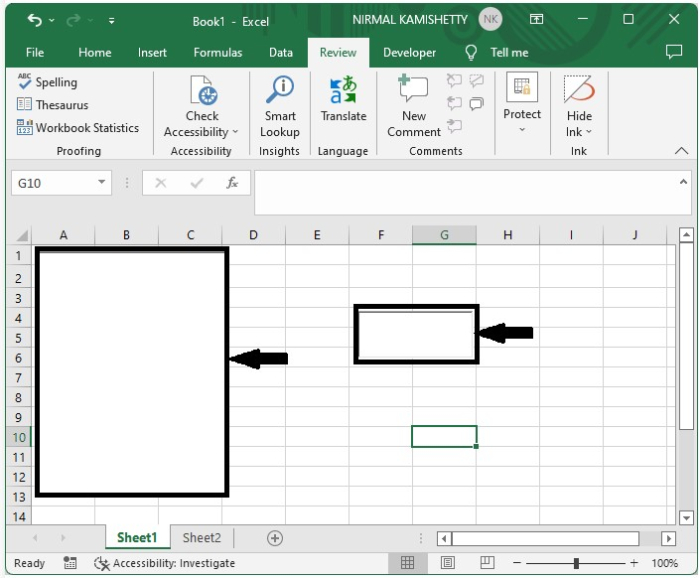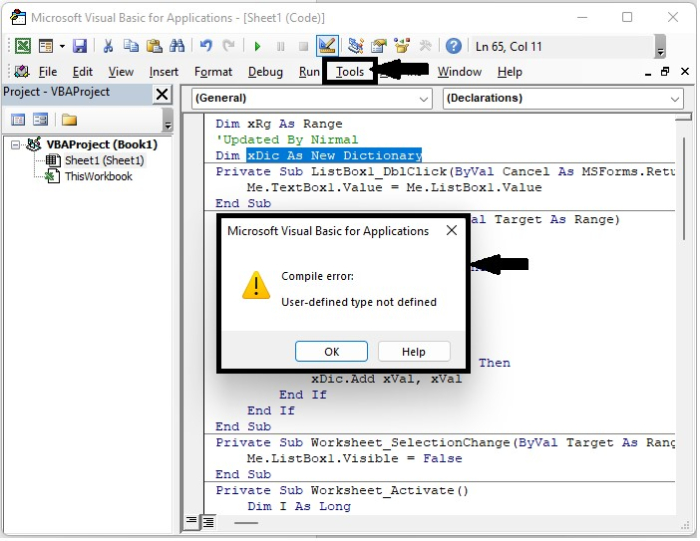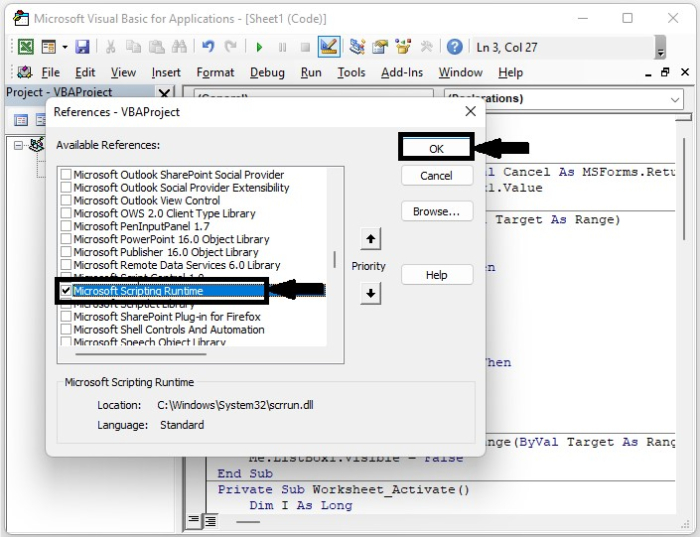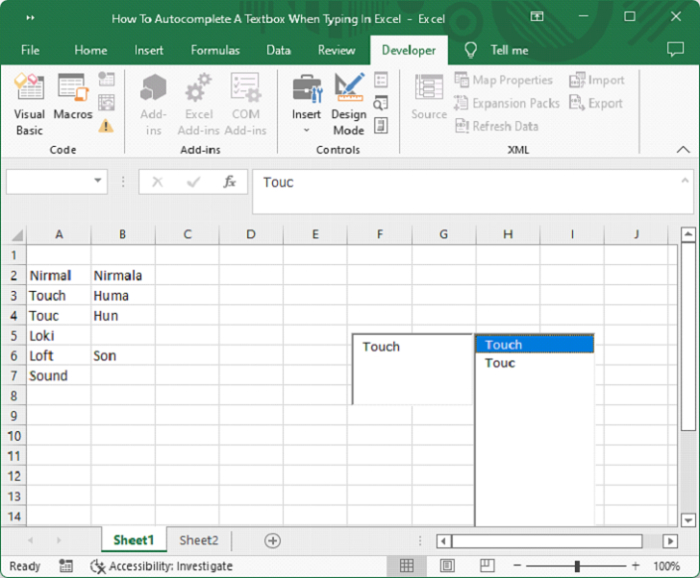# How to Autocomplete a Textbox When Typing in Excel?

We can use auto-complete in Excel to tie the elements in the text box to those that are already present in the list. As this process can’t be completed by default, we need to make some changes to the workbook in order to make the autocomplete possible. Read this tutorial to learn how you can autocomplete a textbox when typing in Excel.

## Autocomplete a Textbox When Typing

Here we will assign a macro to the textbox. Let us see a simple process to understand how we can autocomplete a text box when typing in Excel. We need to use the comment box and list the ActiveX commands.

Step 1

Let us consider a new Excel sheet, and to insert the text box and list box, click on developer, then click on insert, and draw the box boundaries as shown in the below image.Now, right-click on the sheet name and select view code to open the vba application, and type programme into the text box as shown in the below image.

### Program

Dim xRg As Range
'Updated By Nirmal
Dim xDic As New Dictionary
Private Sub ListBox1_DblClick(ByVal Cancel As MSForms.ReturnBoolean)
Me.TextBox1.Value = Me.ListBox1.Value
End Sub
Private Sub Worksheet_Change(ByVal Target As Range)
Dim xVal As String
On Error Resume Next
If IsNumeric(Target.Value) Then
xVal = Str(Target.Value)
Else
xVal = Target.Value
End If
If xVal <> "" Then
If Not xDic.Exists(xVal) Then
End If
End If
End Sub
Private Sub Worksheet_SelectionChange(ByVal Target As Range)
Me.ListBox1.Visible = False
End Sub
Private Sub Worksheet_Activate()
Dim I As Long
Dim xStr As String
On Error Resume Next
If xRg Is Nothing Then
Set xRg = ActiveSheet.UsedRange
End If
Me.ListBox1.Visible = False
xDic.RemoveAll
With Me.ListBox1
For I = 1 To xRg.Count
xStr = xRg(I).Value
If xStr <> "" Then
If Not xDic.Exists(xStr) Then
End If
End If
Next
End With
End Sub
Private Sub TextBox1_KeyUp(ByVal KeyCode As MSForms.ReturnInteger, ByVal Shift As Integer)
With Me.ListBox1
.Top = Me.TextBox1.Top
.Left = Me.TextBox1.Left + Me.TextBox1.Width
.Width = Me.TextBox1.Width
End With
TextBoxVal Me.TextBox1.Object
End Sub
Sub TextBoxVal(xTextBox As Variant)
Dim I As Long
Dim xStr As String
On Error Resume Next
Application.ScreenUpdating = False
If xRg Is Nothing Then Exit Sub
Me.ListBox1.Clear
xStr = xTextBox.Value
If xStr = "" Then
Me.ListBox1.Visible = False
Application.EnableEvents = True
Exit Sub
End If
For I = 0 To UBound(xDic.Items)
If Left(xDic.Items(I), Len(xStr)) = xStr Then
End If
Next
Me.ListBox1.Visible = True
If Me.ListBox1.ListCount > 0 Then
With xTextBox
.Value = Me.ListBox1.List(0)
.SelStart = Len(xStr)
.SelLength = Len(Me.ListBox1.List(0))
End With
End If
Me.ListBox1.Activate
Me.ListBox1.Selected(0) = True
Application.ScreenUpdating = True
End Sub
Private Sub ListBox1_KeyDown(ByVal KeyCode As MSForms.ReturnInteger, ByVal Shift As Integer)
If KeyCode = 13 Then
Me.TextBox1.Value = Me.ListBox1.Value
End If
End SubWe get an error, as shown in the above image. To clear the error, click on Tools and select References to open a pop-up, then select the checkbox for Microsoft Scripting Runtime, as shown in the below image.Step 2

Save the sheet as a macro-enabled template, and type the data in the list of the Excel sheet as shown in the below image.## Conclusion

In this tutorial, we used a simple example to demonstrate how we can autocomplete a textbox when typing in Excel.

Updated on: 03-Jan-2023

337 Views